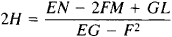# Mean Curvature

Also found in: Wikipedia.

## mean curvature

[¦mēn ′kər·və·chər]
(mathematics)
Half the sum of the principal curvatures at a point on a surface.

## Mean Curvature

The mean curvature of a surface at a given point P on the surface is equal to the average of the principal curvatures of the surface at the point (seeDIFFERENTIAL GEOMETRY). If E, F, and G are the coefficients of the first fundamental quadratic form of the surface and L, M, and N are the coefficients of the surface’s second fundamental quadratic form, then the mean curvature H can be computed from the formulaIf the mean curvature is equal to zero at every point of the surface, then the surface is known as a minimal surface.

Mentioned in ?
References in periodicals archive ?
In doing so it traps some air and, in trying to minimize area, compresses that air inside; this sets up a pressure differential across the surface that enters into the dynamics of surface formation Soap bubbles are not minimal surfaces; they are what mathematicians call surfaces of constant mean curvature. These are formed by processes that try to minimize area, subject to the constraint that a fixed volume must be enclosed.
where [H.sub.s] denotes the mean curvature with respect to the unit normal pointing towards spatial infinity.
Finally we describe the Lagrangian self-similar solutions of the Mean Curvature Flow which are SO(n)-equivariant.
For readers who have completed their study of linear elliptic differential equations and intend to explore nonlinear ones, Han discusses quasilinear and fully nonlinear equations, focusing on two important nonlinear elliptic differentials closely related to geometry, the mean curvature equation and the Monge-Ampere equation.
For instance, in , Carmo and Dajczer define rotational hyper-surfaces with constant mean curvature (cmc) in hyperbolic n-space.
The mean curvature of the bilayer increases gradually (Figure 5) until the stable meander shape form is achieved after approximately 16 [micro]s.
We provide a variational approximation scheme to the anisotropic mean curvature flow; a family [{[[GAMMA].sub.t]}.sub.t[greater than or equal to]0] of closed hyper-surfaces in [R.sup.N] evolving by the following equation
Smyth: A formula of Simons' type and hypersurfaces with constant mean curvature, J.
Among the topics are variation on the p-Laplacian, extremal functions in Poincare-Sobolev inequalities for functions of bounded variation, homocline type solutions for a class of differential equations with periodic coefficients, the cooperative case of quasilinear and singular systems, weighted asymmetric problems for an indefinite elliptic operator, multiple non-trivial solutions of the Dirichlet problem for the prescribed mean curvature equation, and existence of nodal solutions for some nonlinear elliptic problems.
area S = 2[V.sub.2], the integral of mean curvature M = [pi][V.sub.1], and the Euler characteristic X = [V.sub.0], see e.g.
The trace of -[[??].sub.[xi]] is called the lightlike mean curvature [H.sub.[xi]] on M associated with [xi].

Site: Follow: Share:
Open / Close